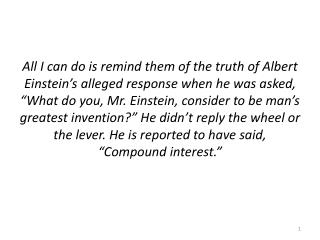DownloadDownload PresentationSavings Accounts

# Savings Accounts

Download Presentation## Savings Accounts

- - - - - - - - - - - - - - - - - - - - - - - - - - - E N D - - - - - - - - - - - - - - - - - - - - - - - - - - -
##### Presentation Transcript

1. All I can do is remind them of the truth of Albert Einstein’s alleged response when he was asked, “What do you, Mr. Einstein, consider to be man’s greatest invention?” He didn’t reply the wheel or the lever. He is reported to have said, “Compound interest.”

2. Savings Accounts Consumer Math 2012-2013

3. Overview • Differences between checking and savings accounts • APR vs. APY • Simple Interest • Compound Interest • Online vs. Physical • Smart Phone

4. Differences • Interest Earned • Savings: All earn interest • Checking: Few earn interest (if you meet requirements) • Number of Transactions • Savings: Unlimited deposits, 3-6 withdrawals a month • Checking: Unlimited deposits/withdrawals • Funds Access • Savings: Limited (in person or online transfers from one account to another) • Checking: Convenient (ATMS, in person, or online) • Fees • Savings: None (if monthly withdrawals don’t go over limit) • Checking: Overdraft fees, minimum balance fees, ATM fees, Online Access fees, Bill Pay fees • Bill Paying • Savings: Not usually offered with online services • Checking: Automatic withdrawals to pay loans, credit cards, gym memberships or other ongoing expenses. Single withdrawals too.

5. Interest • Money you receive from a bank or institution for storing your money (principal) with them • APR (Annual Percentage Rate) • APY (Annual Percentage Yield)

6. APR vs APY • APR • APR is the annual rate of interest without taking into account the compounding of interest within that year. • APY • APY does take into account the effects of intra-year compounding.  Online APR -> APY Calculator http://instacalc.com/4968

7. Example 1 • If your credit card company charges 1% a month APR, what % is that a year?

8. Example 2 • If your credit card company charges 1% a month APY, what % is that a year?

9. Simple Interest A = P + P x R x T • Simple Interest • Money you receive solely based off of a principal amount • A = Final amount • I = Interest Earned • Principal = original invested amount • R = % Rate (as a decimal) • T = Time in years

10. Example 3 • Your bank with pay you 2.1% APR simple interest per year. You deposit \$3,050 in your savings account in January. • How much interest will you have earned in 1 year? • What is the total amount in your savings account?

11. Example 4 • You have a choice between 3 bank accounts. Which one should you choose to make the most interest? • Principal = \$1,200, Rate = 3%, Time = 3 years • Principal = \$1,200, Rate = 2%, Time = 4 years • Principal = \$1,200, Rate = 5%, Time = 2 years

12. Compound Interest • Compound Interest • Money you receive based off of a principal amount and any interest already added on to the principal • Formula for annual compound interest A = final amount P = original invested amount (principal) r = annual % rate (as a decimal) t = number of years n = number of times the interest is compounded per year

13. Principal = \$1,000 & APR = 10% • Simple Interest (Non-compounding): • Compound Interest (Annual Compounding): Interest on \$1000 = \$100 Interest on \$1000 = \$100 Interest on \$1000 = \$100 Year 2 Start Year 3 Start Year 4 Start Year 4 Start Year 3 Start Year 2 Start Year 1 Year 1 \$1,210 \$1,331 \$1,100 \$1,000 \$1,000 \$1,100 \$1,200 \$1,300 Interest on \$1,100 = \$110 Interest on \$1000 = \$100 Interest on \$1,210 = \$121

14. Simple vs. Compound • Principal = \$2,000 • Rate = 5% • Time = 6 years • Compounded Annually Simple InterestCompounded Interest

15. Compound Interest Calculator http://www.ultimatecalculators.com/future_value_annuity_calculator.html • Retirement Time Horizon: • Assumed Interest Rate: • Compounded Annually

16. Example 5 • Calculate the final amount in an account receiving simple interest on an account of \$5,000 at a rate of 5% APR after 5 years.

17. Example 6 • Calculate the final amount in an account receiving compound interest on an account of \$5,000 at a rate of 5% APR compounded annually after 5 years.